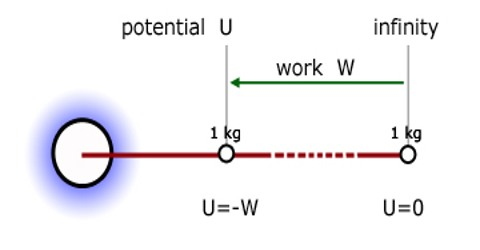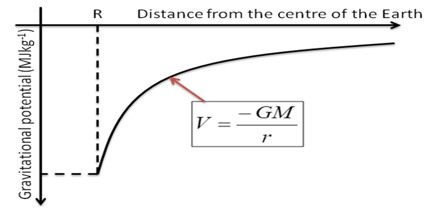# Gravitational Potential

Gravitational Potential

The gravitational potential at a point due to the earth is defined as the amount of work done in moving a unit mass from infinity to that point. It is denoted by V.

So, the gravitational potential of a point in a gravitational field is the work done per unit mass by the pull of gravity to bring a body from infinity to that point. It is to be noted that only attraction force is enough to work on two bodies. No external force is required. So gravitational potential is expressed by negative quantity, means that in any point of gravitational field potential as negative.

It is a scalar quantity and characteristic of a point in a gravitational field whose gradient equals the intensity of the field and equal to the work required to move a body of unit mass from given point to a point infinitely remote.

Unit: In M.K.S. or S.I. unit, its unit is Joule/kilogram or, Jkg-1Gravitational potential is the potential energy per kilogram at a point in a field. So the units are Jkg-1, joules per kilogram.

The equation for potential is: ϕ = – Gm / r

where

G = the universal gravitational constant

m = the mass causing the field

r = the distance between the centre of the mass causing the field and the point you are considering.

Gravitational Potential difference: the amount of work done in moving a unit mass from one point to another point in the gravitational field is called gravitational potential difference.

If the displacement is along the duet bon of gravitational force the potential difference is negative and if the displacement is opposite to the attractive force then it is positive.